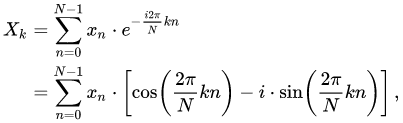# How to Compute a Discrete-Fourier Transform Coefficients Directly in Java?

The Discrete Fourier Transform (DFT) generally varies from 0 to 360. There are basically N-sample DFT, where N is the number of samples. It ranges from n=0 to N-1. We can get the co-efficient by getting the cosine term which is the real part and the sine term which is the imaginary part.

The formula of DFT:Example :

```Input:

Enter the values of simple linear equation
ax+by=c
3
4
5
Enter the k DFT value
2

Output:

(-35.00000000000003) - (-48.17336721649107i)

Input:

Enter the values of simple linear equation
ax+by=c
2
4
5
Enter the k DFT value
4

Output:

(-30.00000000000001) - (-9.747590886987172i)```

Approach:

• First, let us declare the value of N is 10
• We know the formula of DFT sequence is X(k)= e^jw ranges from 0 to N-1
• Now we first take the inputs of a, b, c, and then we try to calculate in “ax+by=c” linear form
• We try to take the function in an array called ‘newvar’.
`newvar[i] = (((a*(double)i) + (b*(double)i)) -c);`
• Now let us take the input variable k, and also declare sin and cosine arrays so that we can calculate real and imaginary parts separately.
`cos[i]=Math.cos((2*i*k*Math.PI)/N);`
`sin[i]=Math.sin((2*i*k*Math.PI)/N);`
• Now let us take real and imaginary variables
• Calculating imaginary variables and real variables like
`real+=newvar[i]*cos[i];`
`img+=newvar[i]*sin[i];`
• Now we will print this output in a+ ib form

Implementation:

## Java

 `// Java program  to Compute a Discrete-Fourier` `// Transform Coefficients Directly`   `import` `java.io.*;` `import` `java.util.Scanner;`   `class` `GFG {`   `    ``public` `static` `void` `main(String[] args)` `    ``{`   `        ``// Size of the N value` `        ``int` `N = ``10``;`   `        ``// Enter the values of simple linear equation` `        ``System.out.println(` `            ``"Enter the values of simple linear equation"``);` `        ``System.out.println(``"ax+by=c"``);`   `        ``// We declare them in data_type double..` `        ``double` `a = ``3.0``;` `        ``double` `b = ``4.0``;` `        ``double` `c = ``5.0``;`   `        ``// Here newvar function array is declared in size` `        ``// N..` `        ``double``[] newvar = ``new` `double``[N];`   `        ``// Now let us loop it over N and take the function` `        ``// Now the newvar array will calculate the function` `        ``// ax+by=c for N times`   `        ``for` `(``int` `i = ``0``; i < N; i++) {` `            ``// This is the way we write that,` `            ``// We are taking array A as of 'a'x` `            ``// array B as of 'b'y` `            ``newvar[i]` `                ``= (((a * (``double``)i) + (b * (``double``)i)) - c);` `        ``}`   `        ``System.out.println(``"Enter the k DFT value"``);`   `        ``// Here we declare the variable k` `        ``int` `k = ``2``;`   `        ``// Here we take 2 terms cos and sin arrays` `        ``// which will be useful to calculate the real and` `        ``// imaginary part The size of both arrays will be 10`   `        ``double``[] cos = ``new` `double``[N];` `        ``double``[] sin = ``new` `double``[N];`   `        ``// Iterating it to N` `        ``// Now let us calculate the formula of cos and sin`   `        ``for` `(``int` `i = ``0``; i < N; i++) {`   `            ``// Here cos term is real part which is` `            ``// multiplied into 2ikpie/N` `            ``cos[i] = Math.cos((``2` `* i * k * Math.PI) / N);`   `            ``// Here sin term is imaginary part which is also` `            ``// multiplied into 2ikpie/N` `            ``sin[i] = Math.sin((``2` `* i * k * Math.PI) / N);` `        ``}`   `        ``// Now to know the value of real and imaginary terms` `        ``// First we declare their respective variables` `        ``double` `real = ``0``, img = ``0``;`   `        ``// Now let us iterate it till N`   `        ``for` `(``int` `i = ``0``; i < N; i++) {`   `            ``// real part  can be calculated by adding it` `            ``// with newvar and multiplying it with cosine` `            ``// array` `            ``real += newvar[i] * cos[i];`   `            ``// Imaginary part is calculated by adding it` `            ``// with newvar and multiplying it with sine` `            ``// array` `            ``img += newvar[i] * sin[i];` `        ``}`   `        ``// Now real and imaginary part can be written in` `        ``// this equation form` `        ``System.out.println(``"("` `+ real + ``") - "` `                           ``+ ``"("` `+ img + ``"i)"``);` `    ``}` `}`

Output

```Enter the values of simple linear equation
ax+by=c
Enter the k DFT value
(-35.00000000000003) - (-48.17336721649107i)```

Time complexity: O(n)

Auxiliary space: O(n) for array

Whether you're preparing for your first job interview or aiming to upskill in this ever-evolving tech landscape, GeeksforGeeks Courses are your key to success. We provide top-quality content at affordable prices, all geared towards accelerating your growth in a time-bound manner. Join the millions we've already empowered, and we're here to do the same for you. Don't miss out - check it out now!

Previous
Next